# AP Calculus AB Practice Test 34

### Test Information9 questions27 minutes

Calculator Allowed

1. Let G(x) = [f (x)]2. At x = a, the graph of f is increasing and concave downward, while G is decreasing. Which describes the graph of G at x = a ?

2. The value of c for which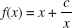has a local minimum at x = 3 is

3. An object moving along a line has velocity v (t) = t cos t - ln (t + 2), where 0 t 10. The object achieves its maximum speed when t is approximately

4.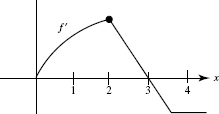The graph of f , which consists of a quarter-circle and two line segments, is shown above. At x = 2, which of the following statements is true?

5. Let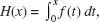where f is the function whose graph appears below.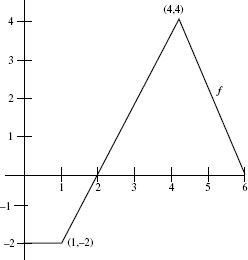The local linearization of H(x) near x = 3 is H(x)6. The table shows the speed of an object, in feet per second, at various times duringa 12-second interval.

 time (sec) 0 3 6 7 8 10 12 speed (ft/sec) 15 14 11 8 7 3 0

Estimate the distance the object travels, using the midpoint method with 3 subintervals.

7. In a marathon, when the winner crosses the finish line many runners are still onthe course, some quite far behind. If the density of runners x miles from the finish line is given by R(x) = 20[1 - cos(1 + 0.03x2)] runners per mile, how many are within 8 miles of the finish line?

8. Which best describes the behavior of the function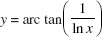at x = 1?

9. If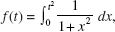then f (t) equals